Скачать презентацию CHAPTER 2 Gravitation and the Waltz of the

f3fa8b40efb5077537f67a3d762a6a21.ppt

• Количество слайдов: 34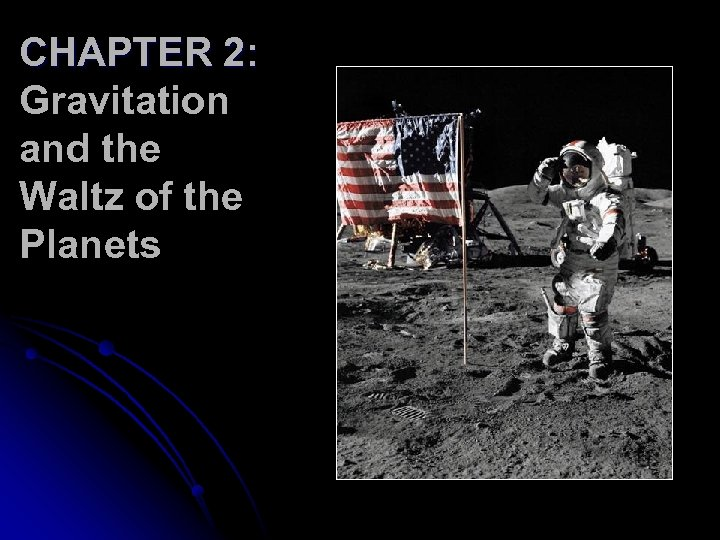CHAPTER 2: Gravitation and the Waltz of the Planets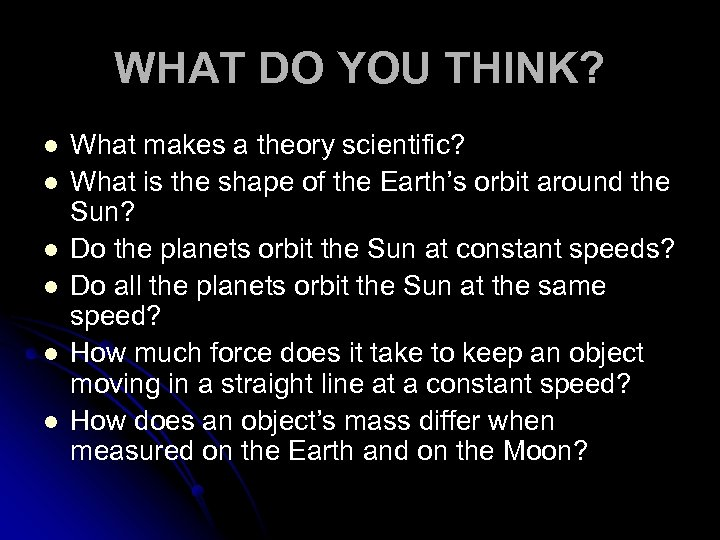WHAT DO YOU THINK? l l l What makes a theory scientific? What is the shape of the Earth’s orbit around the Sun? Do the planets orbit the Sun at constant speeds? Do all the planets orbit the Sun at the same speed? How much force does it take to keep an object moving in a straight line at a constant speed? How does an object’s mass differ when measured on the Earth and on the Moon?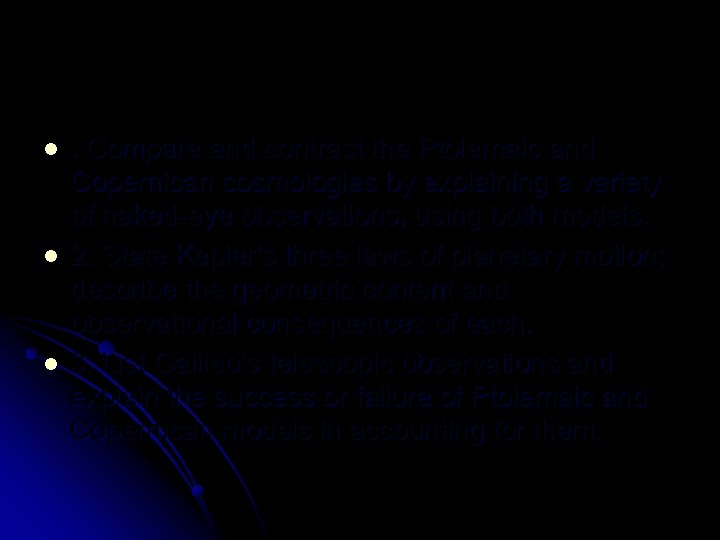l l l . Compare and contrast the Ptolemaic and Copernican cosmologies by explaining a variety of naked-eye observations, using both models. 2. State Kepler’s three laws of planetary motion; describe the geometric content and observational consequences of each. 3. List Galileo’s telescopic observations and explain the success or failure of Ptolemaic and Copernican models in accounting for them.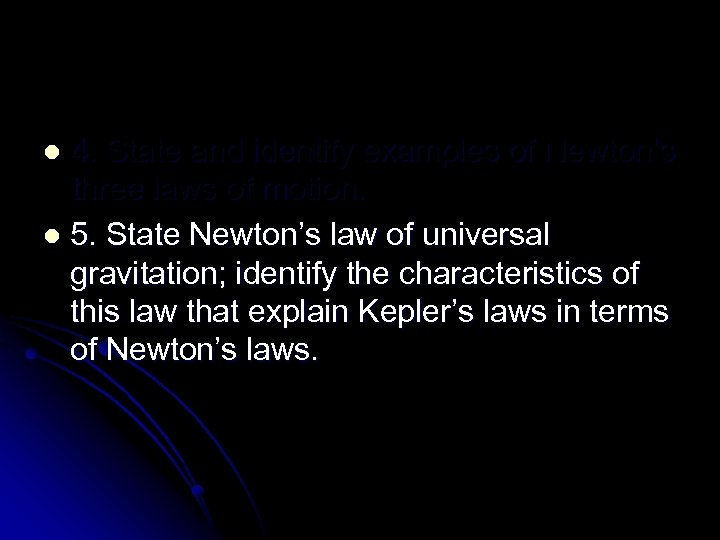4. State and identify examples of Newton’s three laws of motion. l 5. State Newton’s law of universal gravitation; identify the characteristics of this law that explain Kepler’s laws in terms of Newton’s laws. l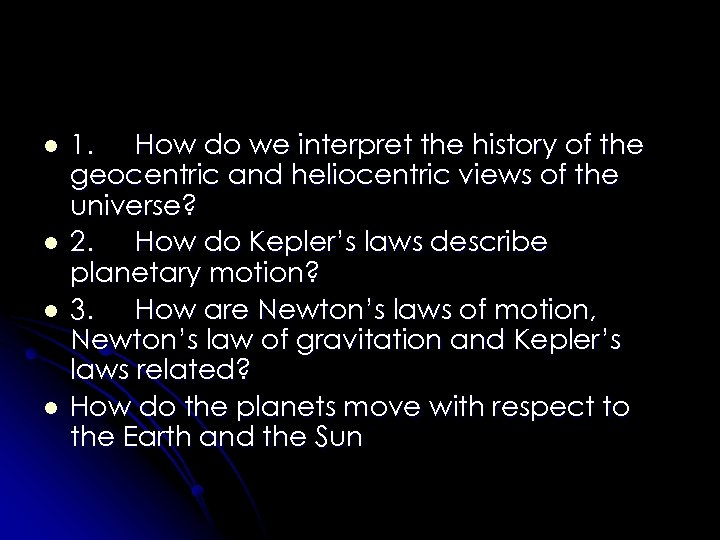l l 1. How do we interpret the history of the geocentric and heliocentric views of the universe? 2. How do Kepler’s laws describe planetary motion? 3. How are Newton’s laws of motion, Newton’s law of gravitation and Kepler’s laws related? How do the planets move with respect to the Earth and the Sun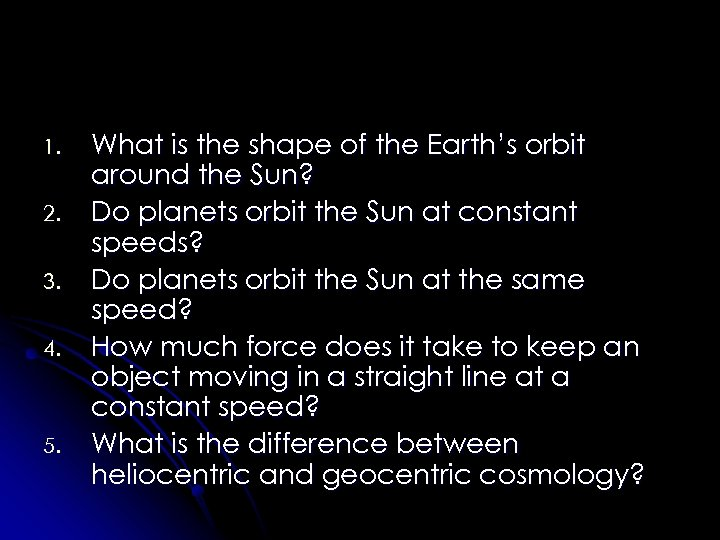1. 2. 3. 4. 5. What is the shape of the Earth’s orbit around the Sun? Do planets orbit the Sun at constant speeds? Do planets orbit the Sun at the same speed? How much force does it take to keep an object moving in a straight line at a constant speed? What is the difference between heliocentric and geocentric cosmology?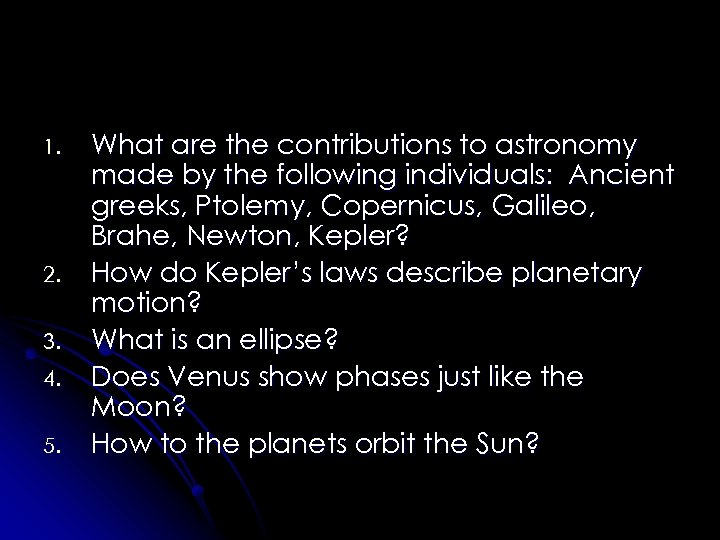1. 2. 3. 4. 5. What are the contributions to astronomy made by the following individuals: Ancient greeks, Ptolemy, Copernicus, Galileo, Brahe, Newton, Kepler? How do Kepler’s laws describe planetary motion? What is an ellipse? Does Venus show phases just like the Moon? How to the planets orbit the Sun?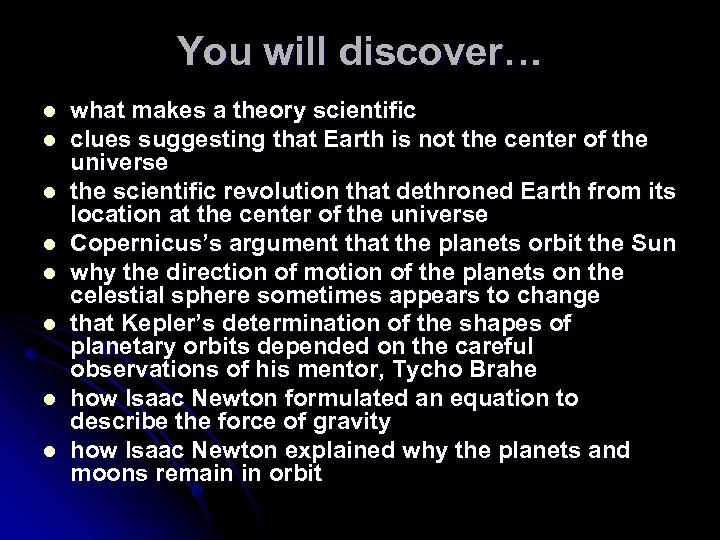You will discover… l l l l what makes a theory scientific clues suggesting that Earth is not the center of the universe the scientific revolution that dethroned Earth from its location at the center of the universe Copernicus’s argument that the planets orbit the Sun why the direction of motion of the planets on the celestial sphere sometimes appears to change that Kepler’s determination of the shapes of planetary orbits depended on the careful observations of his mentor, Tycho Brahe how Isaac Newton formulated an equation to describe the force of gravity how Isaac Newton explained why the planets and moons remain in orbit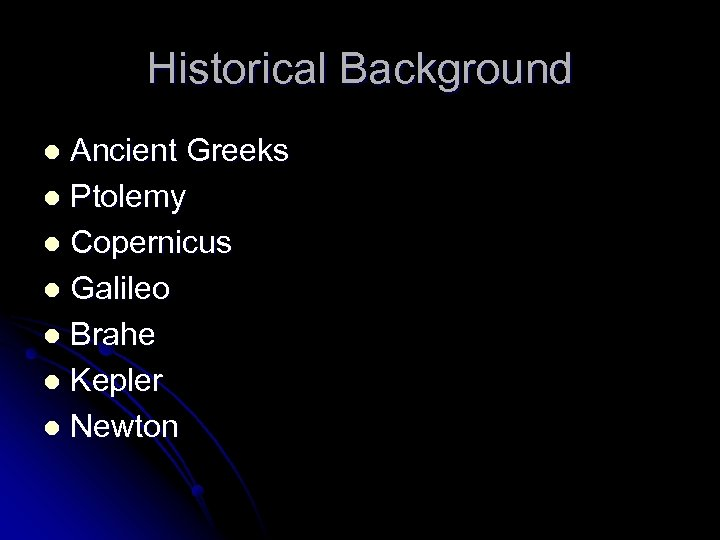Historical Background Ancient Greeks l Ptolemy l Copernicus l Galileo l Brahe l Kepler l Newton l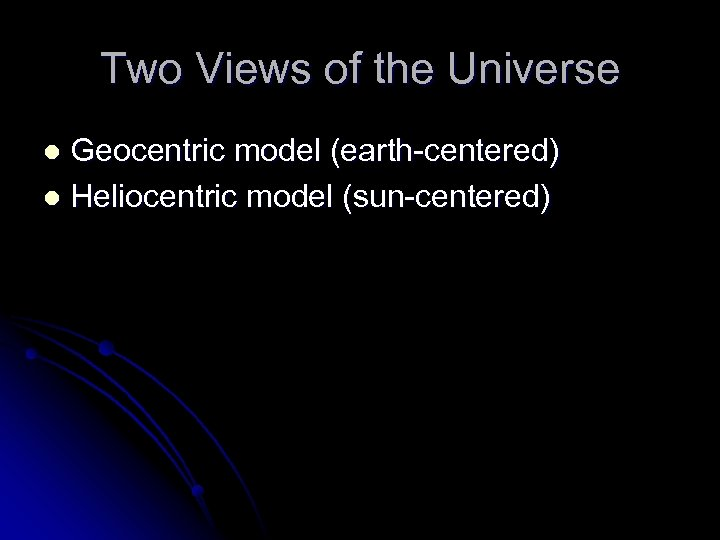Two Views of the Universe Geocentric model (earth-centered) l Heliocentric model (sun-centered) l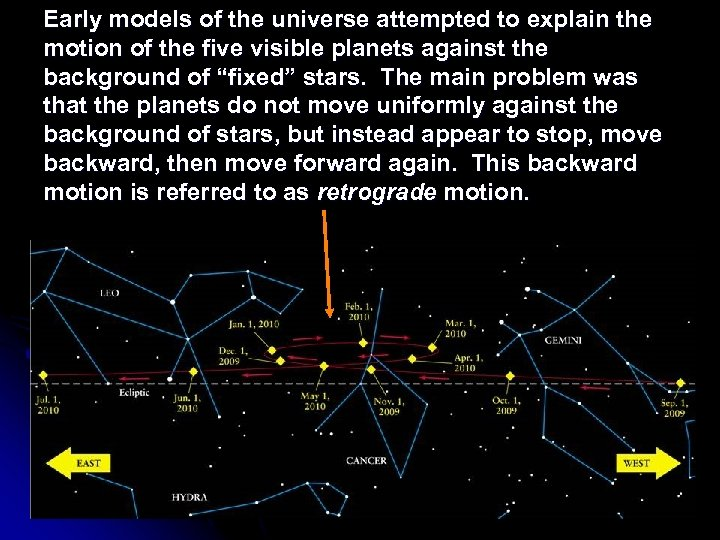Early models of the universe attempted to explain the motion of the five visible planets against the background of “fixed” stars. The main problem was that the planets do not move uniformly against the background of stars, but instead appear to stop, move backward, then move forward again. This backward motion is referred to as retrograde motion.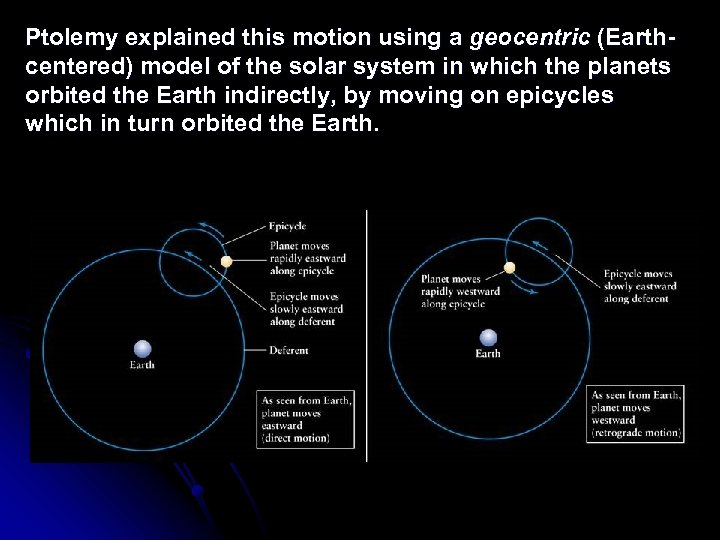Ptolemy explained this motion using a geocentric (Earthcentered) model of the solar system in which the planets orbited the Earth indirectly, by moving on epicycles which in turn orbited the Earth.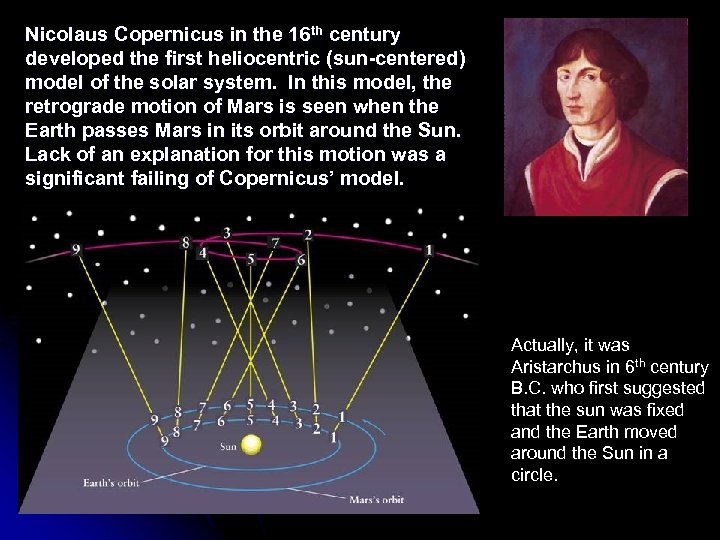Nicolaus Copernicus in the 16 th century developed the first heliocentric (sun-centered) model of the solar system. In this model, the retrograde motion of Mars is seen when the Earth passes Mars in its orbit around the Sun. Lack of an explanation for this motion was a significant failing of Copernicus’ model. Actually, it was Aristarchus in 6 th century B. C. who first suggested that the sun was fixed and the Earth moved around the Sun in a circle.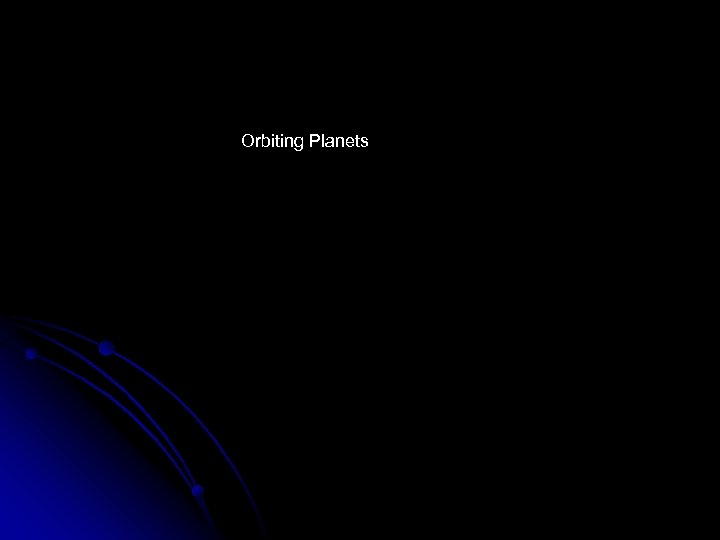Orbiting Planets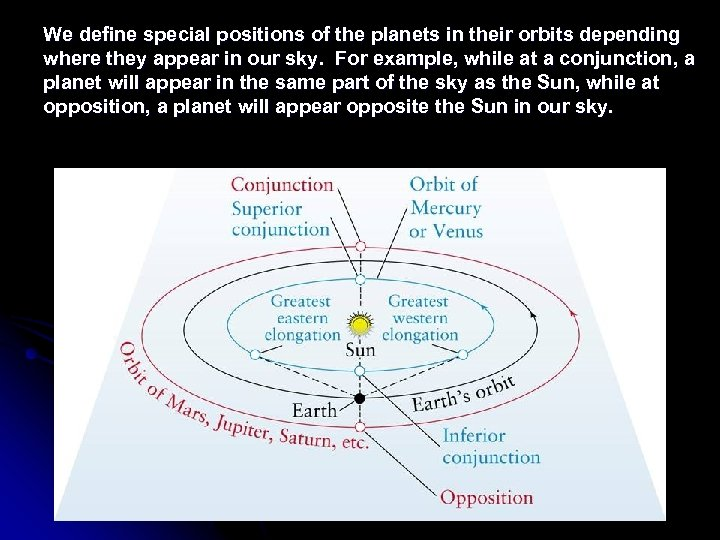We define special positions of the planets in their orbits depending where they appear in our sky. For example, while at a conjunction, a planet will appear in the same part of the sky as the Sun, while at opposition, a planet will appear opposite the Sun in our sky.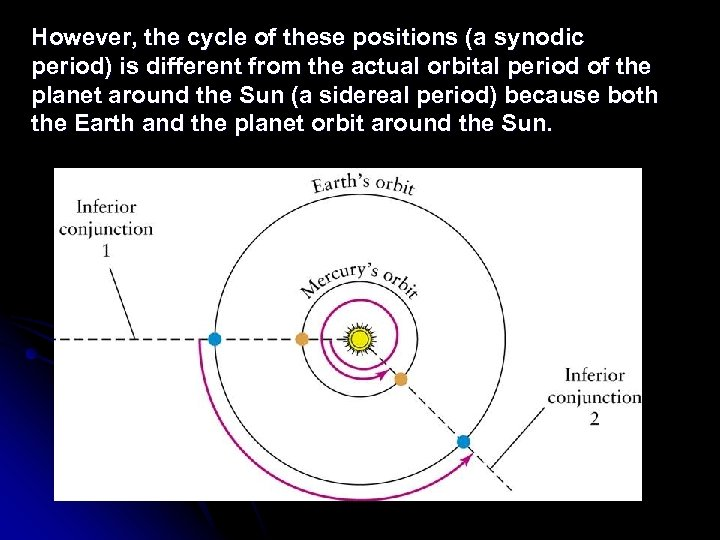However, the cycle of these positions (a synodic period) is different from the actual orbital period of the planet around the Sun (a sidereal period) because both the Earth and the planet orbit around the Sun.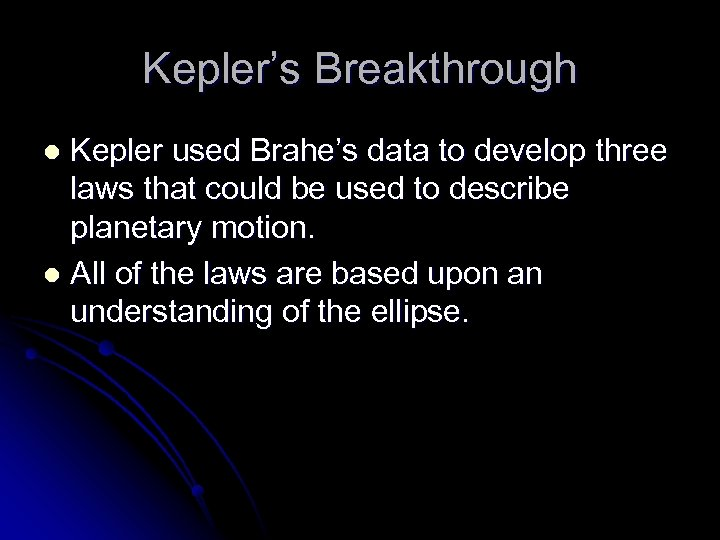Kepler’s Breakthrough Kepler used Brahe’s data to develop three laws that could be used to describe planetary motion. l All of the laws are based upon an understanding of the ellipse. l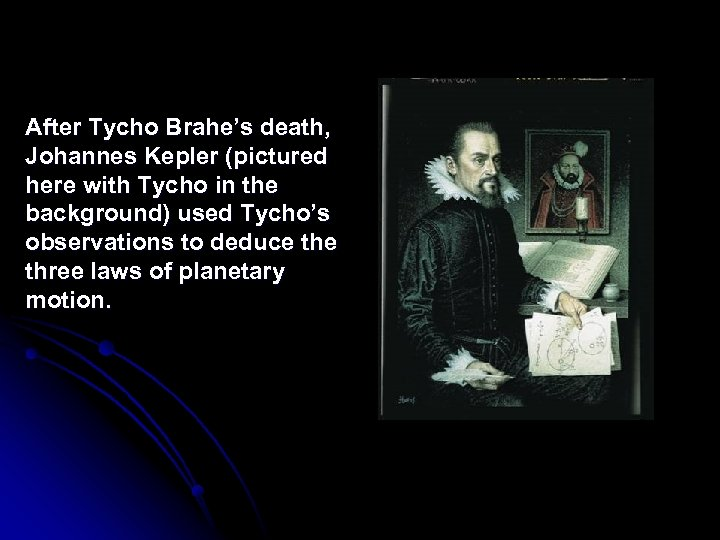After Tycho Brahe’s death, Johannes Kepler (pictured here with Tycho in the background) used Tycho’s observations to deduce three laws of planetary motion.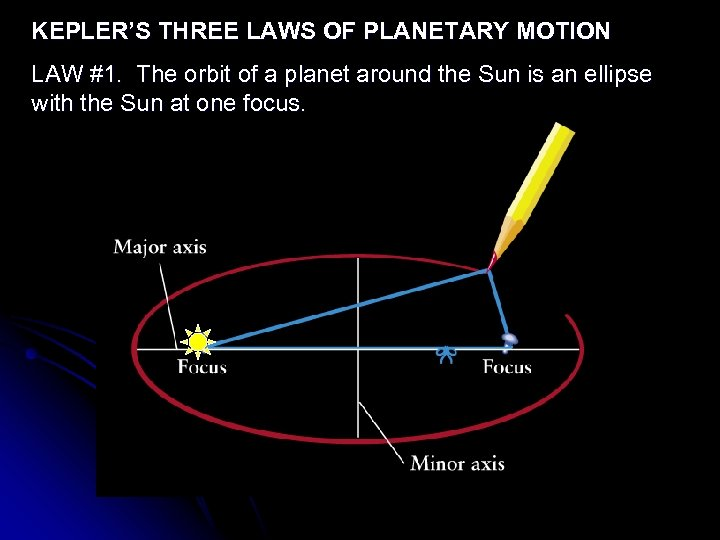KEPLER’S THREE LAWS OF PLANETARY MOTION LAW #1. The orbit of a planet around the Sun is an ellipse with the Sun at one focus.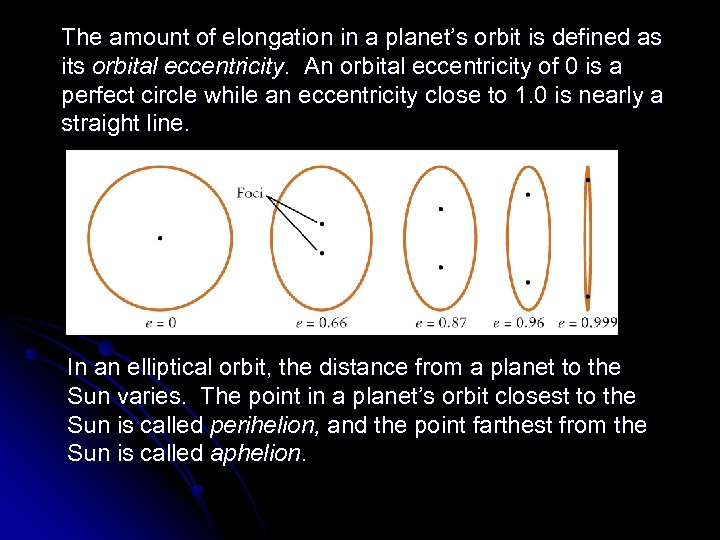The amount of elongation in a planet’s orbit is defined as its orbital eccentricity. An orbital eccentricity of 0 is a perfect circle while an eccentricity close to 1. 0 is nearly a straight line. In an elliptical orbit, the distance from a planet to the Sun varies. The point in a planet’s orbit closest to the Sun is called perihelion, and the point farthest from the Sun is called aphelion.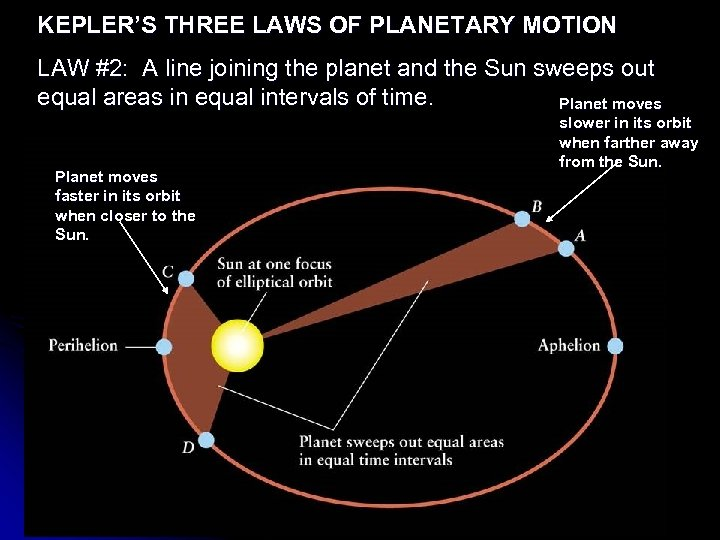KEPLER’S THREE LAWS OF PLANETARY MOTION LAW #2: A line joining the planet and the Sun sweeps out equal areas in equal intervals of time. Planet moves faster in its orbit when closer to the Sun. slower in its orbit when farther away from the Sun.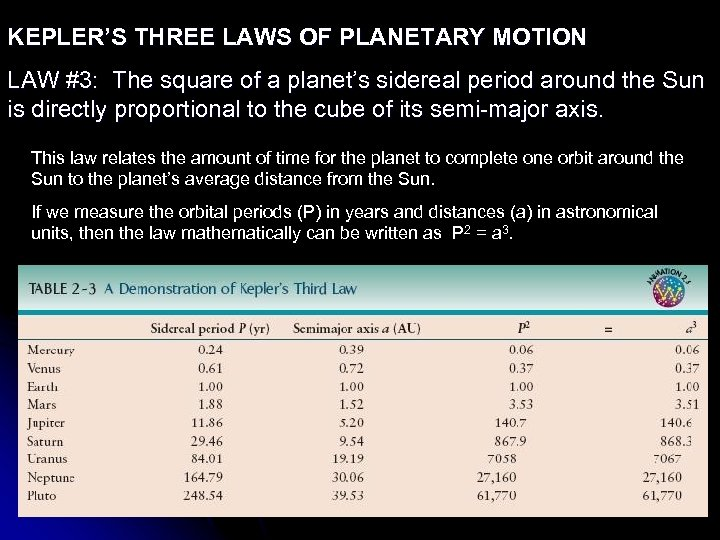KEPLER’S THREE LAWS OF PLANETARY MOTION LAW #3: The square of a planet’s sidereal period around the Sun is directly proportional to the cube of its semi-major axis. This law relates the amount of time for the planet to complete one orbit around the Sun to the planet’s average distance from the Sun. If we measure the orbital periods (P) in years and distances (a) in astronomical units, then the law mathematically can be written as P 2 = a 3.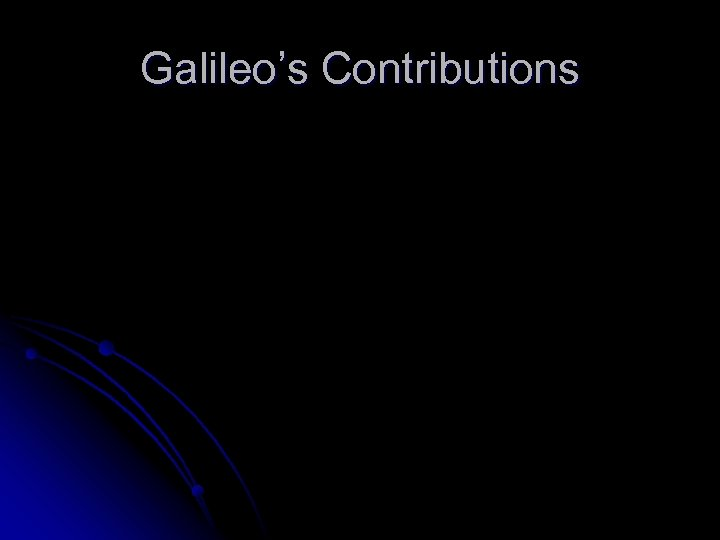Galileo’s Contributions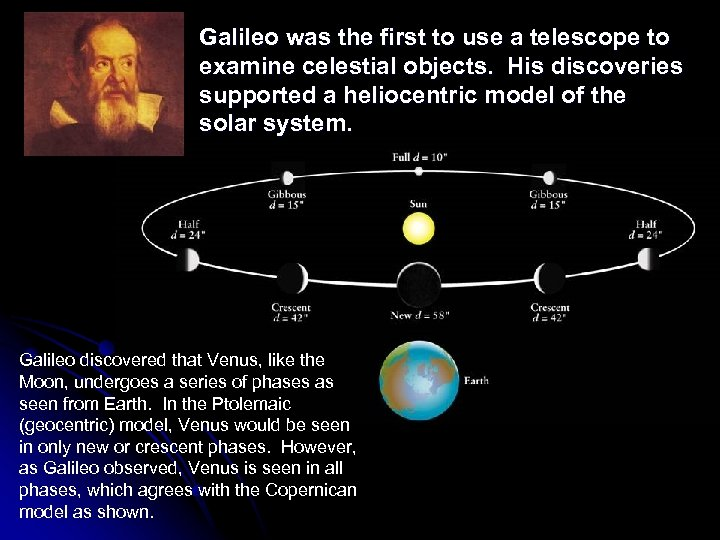Galileo was the first to use a telescope to examine celestial objects. His discoveries supported a heliocentric model of the solar system. Galileo discovered that Venus, like the Moon, undergoes a series of phases as seen from Earth. In the Ptolemaic (geocentric) model, Venus would be seen in only new or crescent phases. However, as Galileo observed, Venus is seen in all phases, which agrees with the Copernican model as shown.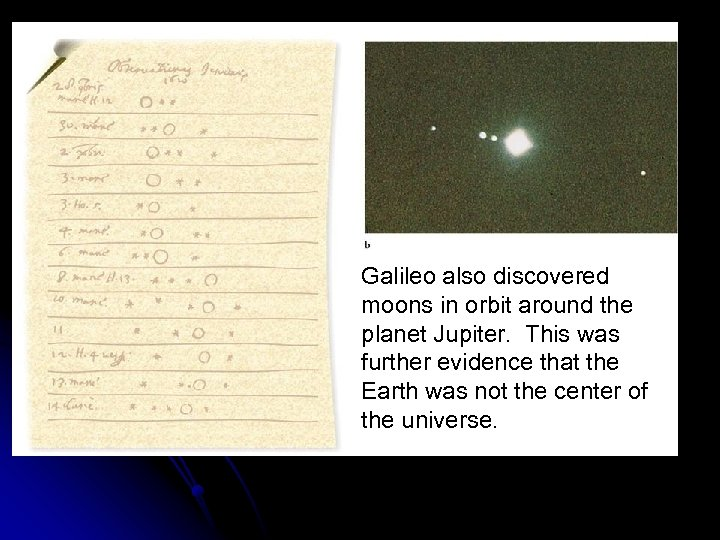Galileo also discovered moons in orbit around the planet Jupiter. This was further evidence that the Earth was not the center of the universe.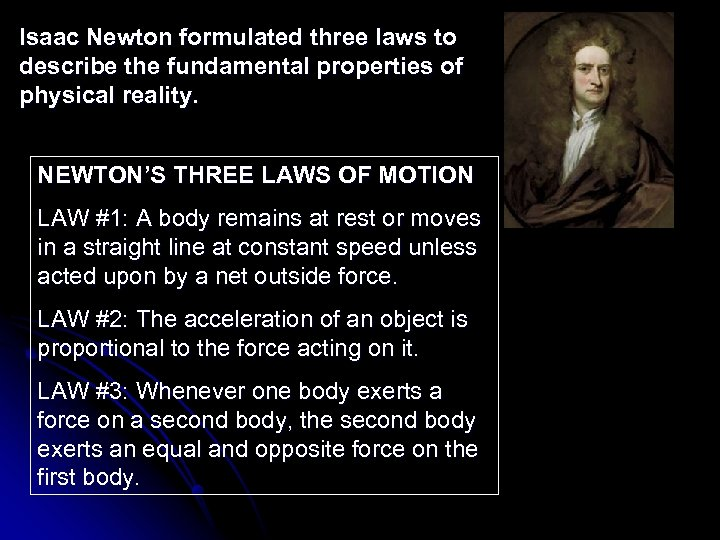Isaac Newton formulated three laws to describe the fundamental properties of physical reality. NEWTON’S THREE LAWS OF MOTION LAW #1: A body remains at rest or moves in a straight line at constant speed unless acted upon by a net outside force. LAW #2: The acceleration of an object is proportional to the force acting on it. LAW #3: Whenever one body exerts a force on a second body, the second body exerts an equal and opposite force on the first body.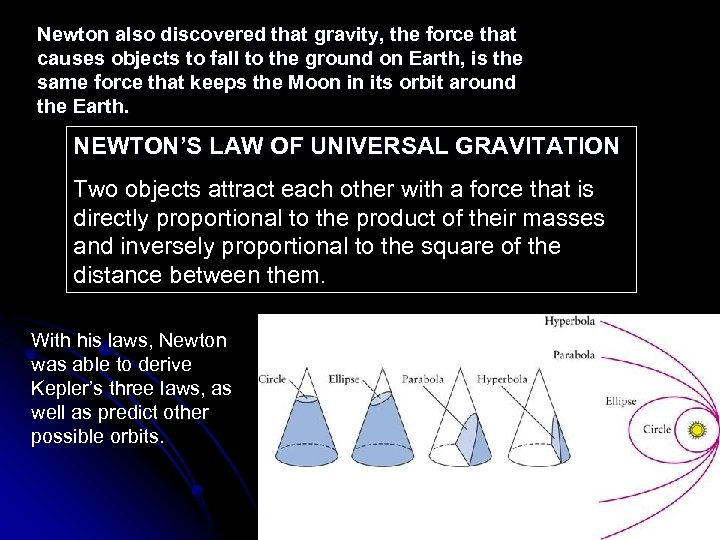Newton also discovered that gravity, the force that causes objects to fall to the ground on Earth, is the same force that keeps the Moon in its orbit around the Earth. NEWTON’S LAW OF UNIVERSAL GRAVITATION Two objects attract each other with a force that is directly proportional to the product of their masses and inversely proportional to the square of the distance between them. With his laws, Newton was able to derive Kepler’s three laws, as well as predict other possible orbits.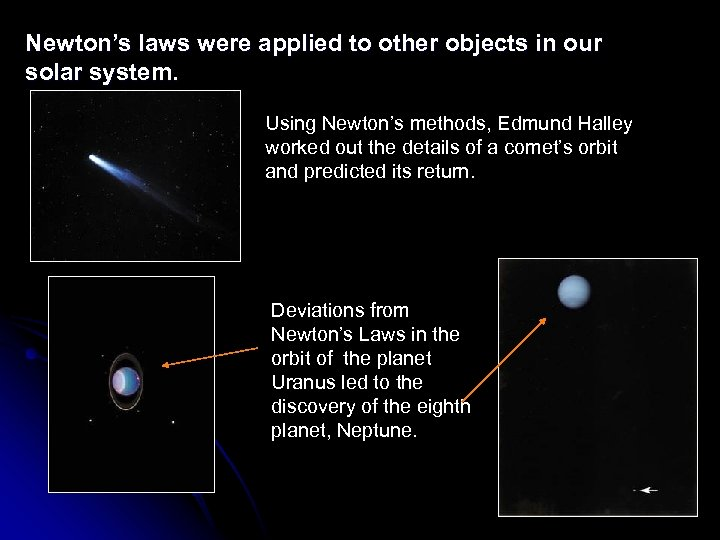Newton’s laws were applied to other objects in our solar system. Using Newton’s methods, Edmund Halley worked out the details of a comet’s orbit and predicted its return. Deviations from Newton’s Laws in the orbit of the planet Uranus led to the discovery of the eighth planet, Neptune.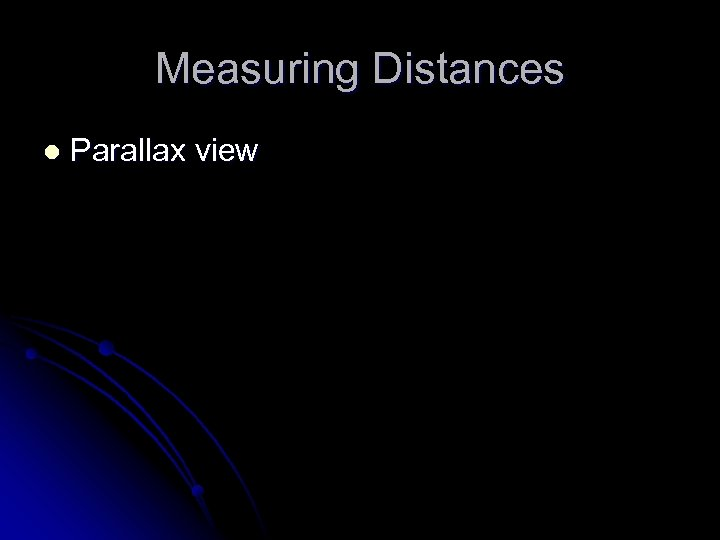Measuring Distances l Parallax view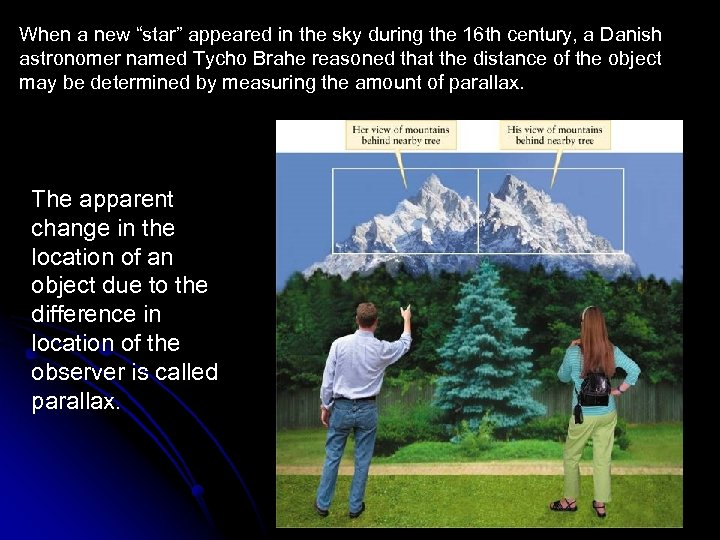When a new “star” appeared in the sky during the 16 th century, a Danish astronomer named Tycho Brahe reasoned that the distance of the object may be determined by measuring the amount of parallax. The apparent change in the location of an object due to the difference in location of the observer is called parallax.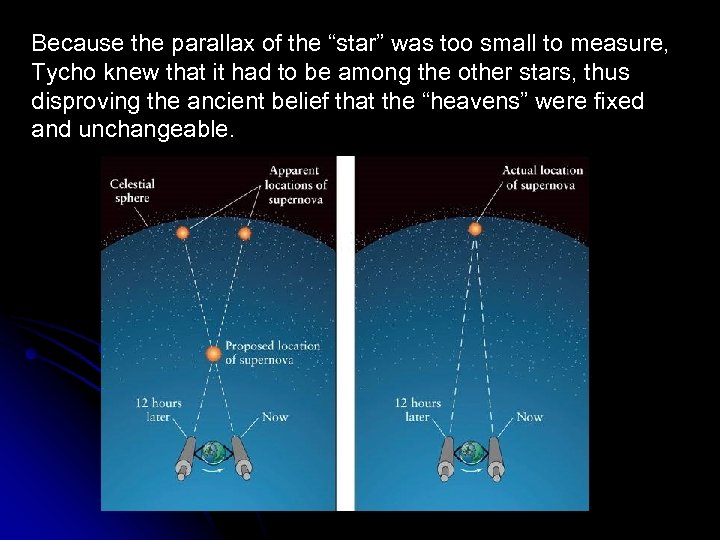Because the parallax of the “star” was too small to measure, Tycho knew that it had to be among the other stars, thus disproving the ancient belief that the “heavens” were fixed and unchangeable.WHAT DID YOU THINK? l l l What makes a theory scientific? If it makes predictions that can be objectively tested and potentially disproved. What is the shape of the Earth’s orbit around the Sun? Elliptical Do the planets orbit the Sun at constant speeds? The closer a planet is to the Sun in its orbit, the faster it is moving. It moves fastest at perihelion and slowest at aphelion.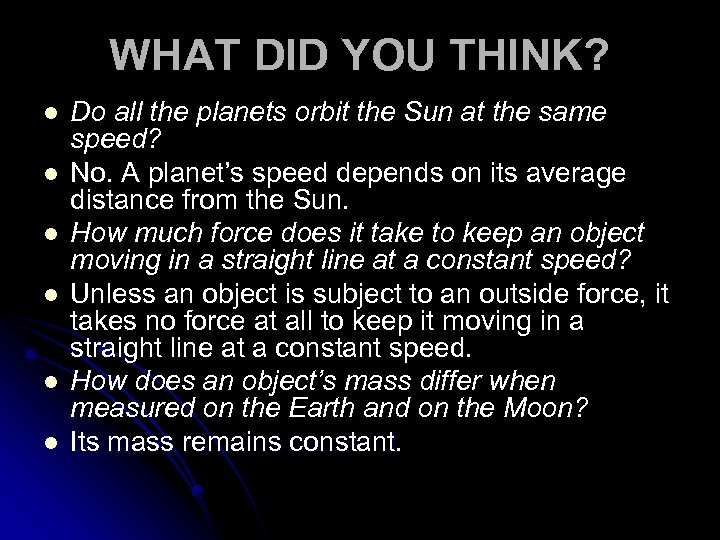WHAT DID YOU THINK? l l l Do all the planets orbit the Sun at the same speed? No. A planet’s speed depends on its average distance from the Sun. How much force does it take to keep an object moving in a straight line at a constant speed? Unless an object is subject to an outside force, it takes no force at all to keep it moving in a straight line at a constant speed. How does an object’s mass differ when measured on the Earth and on the Moon? Its mass remains constant.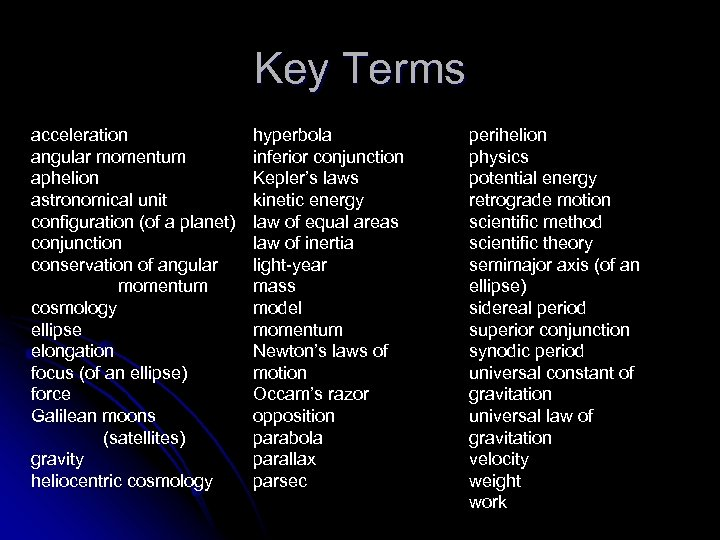Key Terms acceleration angular momentum aphelion astronomical unit configuration (of a planet) conjunction conservation of angular momentum cosmology ellipse elongation focus (of an ellipse) force Galilean moons (satellites) gravity heliocentric cosmology hyperbola inferior conjunction Kepler’s laws kinetic energy law of equal areas law of inertia light-year mass model momentum Newton’s laws of motion Occam’s razor opposition parabola parallax parsec perihelion physics potential energy retrograde motion scientific method scientific theory semimajor axis (of an ellipse) sidereal period superior conjunction synodic period universal constant of gravitation universal law of gravitation velocity weight work